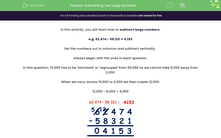# Subtract Two Large Numbers Using Column Method and Exchanging

In this worksheet, students will learn and practise how to subtract two large numbers using the formal column method.Key stage:  KS 2

Curriculum topic:   Number: Addition and Subtraction

Curriculum subtopic:   Add/Subtract Four-Digit (or more) Numbers

Popular topics:   Subtraction worksheets

Difficulty level:#### Worksheet Overview

In this activity, we will look at how to subtract large numbers.

Example

62,474 - 58,321 = 4,153

Set the numbers out in columns and subtract vertically.

Always begin with the ones column in each calculation.

In this example, 10,000 has to be 'exchanged' or 'regrouped' from 60,000 as we cannot take 8,000 away from 2,000. This is also known as 'borrowing'.

When we carry across 10,000 to 2,000 we then create 12,000.

12,000 - 8,000 = 4,000We will now solve a series of calculations that require column subtraction.### What is EdPlace?

We're your National Curriculum aligned online education content provider helping each child succeed in English, maths and science from year 1 to GCSE. With an EdPlace account you’ll be able to track and measure progress, helping each child achieve their best. We build confidence and attainment by personalising each child’s learning at a level that suits them.

Get started